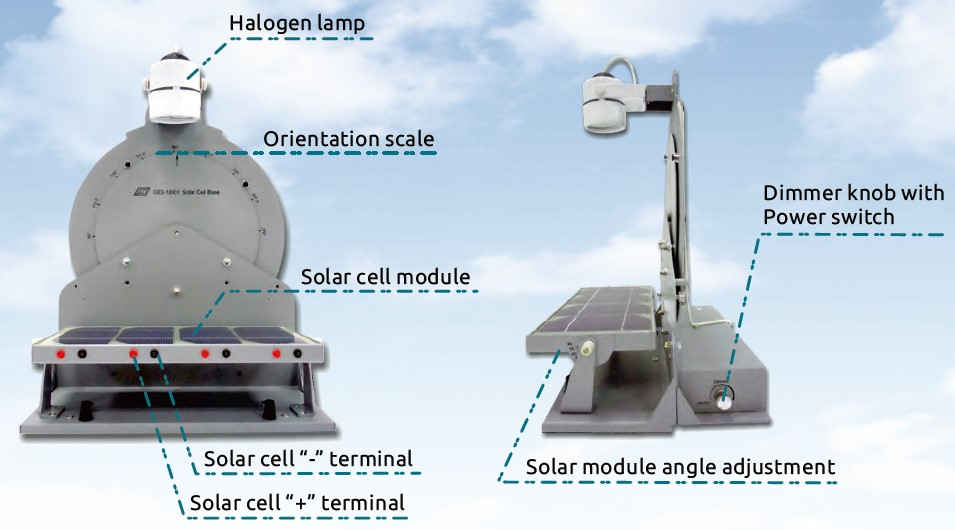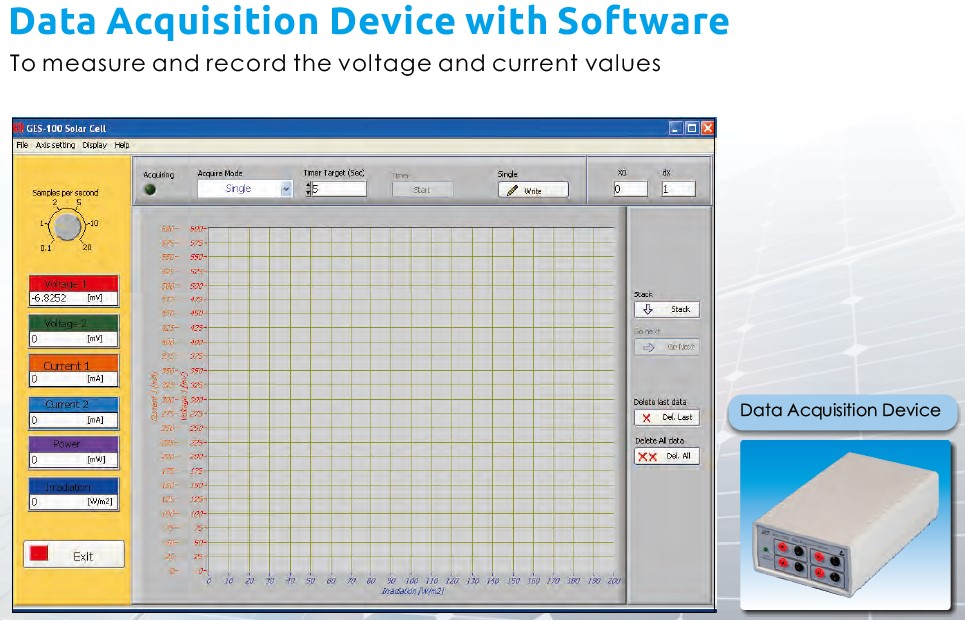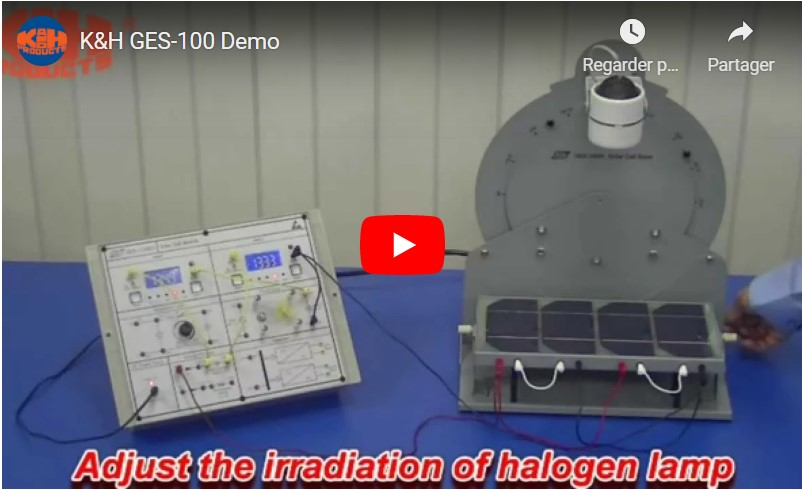# K&H GES-100 Solar Cell Trainer

The GES-100 Solar Cell Trainer is an easy and self-contained trainer designed for learning the basic configuration and characteristics of a solar cell.

## Description

Solar Cell Trainer

The GES-100 Solar Cell Trainer is an easy and self-contained trainer designed for learning the basic configuration and characteristics of a solar cell.

Through the use of different irradiations for various load units, students study the photoelectric effect of solar cells and plot the current-voltage curve as well as charging / discharging curvesList of experiments

• Measuring the irradiation of various light sources
• Energy conversion of solar cells
• Diode characteristic of a solar cell
• Effect of light-sensing area on the open-circuit voltage of solar cell
• Effect of light-sensing area on the short-circuit current of solar cell
• Effect of irradiation on open-circuit voltage and short-circuit current of solar cells
• Relationship between the angle of irradiation and the short-circuit current of solar cell
• Open-circuit voltage and short-circuit current of solar cells connected in series-shading
• Open-circuit voltage and short-circuit current of solar